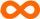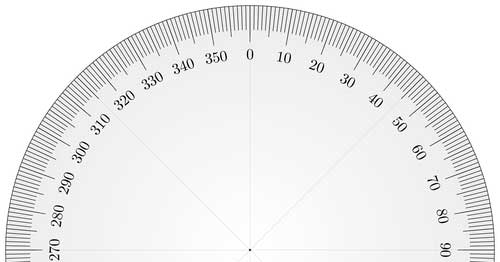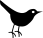Why 12 Dozen and 360 Degrees Makes Sense

Math students through the ages pound their heads on their desks, asking the same question: Why do the numbers 12, 60, and 360 show up so often? Specifically:

• 12 inches in a foot; 360 inches in 30 feet
• 12 is one dozen; 12 dozen is a gross
• 60 seconds in a minute
• 60 minutes in an hour (so 3600 seconds per hour)
• 360 degrees in a circle

Anyone who uses metric measurements immediately finds these sorts of numbers cumbersome and bizarre. Sure, these numbers are a hassle if you’re multiplying, adding, or subtracting. But in one respect these numbers work far better than metric: dividing equally.

For example, 12 can be divided equally into halves, thirds, fourths, and sixths. So if you’re selling eggs, a group of a dozen makes far more sense than a group of ten.

Similarly, it’s easy to divide 60 seconds into 2, 3, 4, 5, 6, 10, 12, 15, 20, or 30 parts.

And dividing a circle into 360 degrees makes sense because 360 can be evenly split into 2, 3, 4, 5, 6, 8, 9, 10, 12, 15, 18, 20, 24, 30, and so on…

100 degrees is wonderful when you need to work with fine increments (such as the difference between 35 and 36 degrees Celsius) but it’s a pain when you need to split it into thirds, eighths, and other odd divisions.

•Spectrums